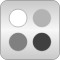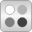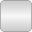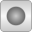# Incompressible

Medium model for T-dependent properties, defined by tables or polynomials# Package ContentsExamples Examples for incompressible mediaCommon Common data structuresTableBased Incompressible medium properties based on tables

# Information

This information is part of the Modelica Standard Library maintained by the Modelica Association.

#### Incompressible media package

This package provides a structure and examples of how to create simple medium models of incompressible fluids, meaning fluids with very little pressure influence on density. The medium properties is typically described in terms of tables, functions or polynomial coefficients.

#### Definitions

The common meaning of incompressible is that properties like density and enthalpy are independent of pressure. Thus properties are conveniently described as functions of temperature, e.g., as polynomials density(T) and cp(T). However, enthalpy can not be independent of pressure since h = u - p/d. For liquids it is anyway common to neglect this dependence since for constant density the neglected term is (p - p0)/d, which in comparison with cp is very small for most liquids. For water, the equivalent change of temperature to increasing pressure 1 bar is 0.025 Kelvin.

Two Boolean flags are used to choose how enthalpy and inner energy is calculated:

• enthalpyOfT=true, means assuming that enthalpy is only a function of temperature, neglecting the pressure dependent term.
• singleState=true, means also neglect the pressure influence on inner energy, which makes all medium properties pure functions of temperature.

The default setting for both these flags is true, which enables the simulation tool to choose temperature as the only medium state and avoids non-linear equation systems, see the section about Static state selection in the Modelica.Media User's Guide.

#### Contents

Currently, the package contains the following parts:

A few examples are given in the Examples package. The model Examples.Glycol47 shows how the medium models can be used. For more realistic examples of how to implement volume models with medium properties look in the Medium usage section of the User's Guide.